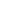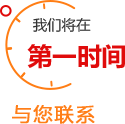## 婴幼用品品牌热榜

• 最新榜单
• 正在投票
• 热榜推荐

• 排名
• 品牌名
• 得票数

• 排名
• 品牌名
• 得票数

• 排名
• 品牌名
• 得票数

• 排名
• 品牌名
• 得票数

• 排名
• 品牌名
• 得票数

• 排名
• 品牌名
• 得票数

• 排名
• 品牌名
• 得票数

• 排名
• 品牌名
• 得票数

• 排名
• 品牌名
• 得票数

• 排名
• 品牌名
• 得票数

• 排名
• 品牌名
• 得票数

• 排名
• 品牌名
• 得票数

• 排名
• 品牌名
• 得票数

• 排名
• 品牌名
• 得票数

• 排名
• 品牌名
• 得票数

• 排名
• 品牌名
• 得票数

• 排名
• 品牌名
• 得票数

• 排名
• 品牌名
• 得票数

• 排名
• 品牌名
• 得票数

• 排名
• 品牌名
• 得票数

• 排名
• 品牌名
• 得票数

• 排名
• 品牌名
• 得票数

• 排名
• 品牌名
• 得票数

• 排名
• 品牌名
• 得票数

• 排名
• 品牌名
• 得票数

• 排名
• 品牌名
• 得票数

• 排名
• 品牌名
• 得票数

• 排名
• 品牌名
• 得票数

• 排名
• 品牌名
• 得票数

• 排名
• 品牌名
• 得票数

• 排名
• 品牌名
• 得票数

• 排名
• 品牌名
• 得票数

• 排名
• 品牌名
• 得票数

• 排名
• 品牌名
• 得票数

• 排名
• 品牌名
• 得票数

• 排名
• 品牌名
• 得票数

• 排名
• 品牌名
• 得票数

• 排名
• 品牌名
• 得票数

• 排名
• 品牌名
• 得票数

• 排名
• 品牌名
• 得票数

• 排名
• 品牌名
• 得票数

• 排名
• 品牌名
• 得票数

• 排名
• 品牌名
• 得票数

• 排名
• 品牌名
• 得票数
• 1
• 3M
• 13851

• 排名
• 品牌名
• 得票数

• 排名
• 品牌名
• 得票数

• 排名
• 品牌名
• 得票数

• 排名
• 品牌名
• 得票数

### 免费上榜*品牌名:

*姓名:

*手机号码:

*留 言:

### 上榜优势

#### 3、精准用户027-87873688

027-87873688通话完全免费，请放心接听！